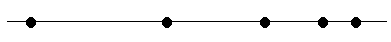Hello there, i have a question that confuses me a lot. Is there a difference between the way that we think about time in many classical physics concepts as if it goes to a negative number. For example, we have dv/dt and we can be sure if velocity increases with respect to time and respect to our inertial frame that the acceleration is non zero (positive or negative). If i plug -dt and i dont know if there is a such thing, velocity becomes negative and i can image the motion like a trajectory starts to reverse its motion. But in the dx^2/d^2t the acc doesnt change its sign. What does it mean? Could we think that if time goes to positive or negative, shouldnt it be the same ? Thank you

Dale
Mentor
2021 Award
But in the dx^2/d^2t the acc doesnt change its sign. What does it mean? Could we think that if time goes to positive or negative, shouldnt it be the same ?
This is the correct one. Acceleration is second order in time: ##a=\frac{d^2}{dt^2}x##. So acceleration is unchanged under time reversal. And therefore force is also unchanged.

In contrast velocity is first order in time: ##v=\frac{d}{dt}x##. So velocity is reversed under time reversal.

That means that the laws of physics* (which describe the forces) are the same under time reversal, and what changes are some of the initial conditions.

*Excluding thermodynamics

Last edited:
•universal2013
Mister T
Gold Member
Imagine a particle moving towards the right and slowing down. Suppose you have a strobe light flashing at regular intervals of time. Each time the strobe light flashes it reveals the location of the particle. The dots in the following drawing show those locations:Let's say the velocity is positive, which would make the acceleration negative. If you reverse time you see that the particle would move to the left, speeding up. Now the velocity is negative but the acceleration is also negative! Note that in both cases the acceleration is directed to the left, and so is the net force.

As @Dale says, the acceleration and the force are unchanged under time reversal.

Also, note that when you make ##dt## negative you are not necessarily making ##t## negative. That is, having time go backwards is not the same thing as time being negative.

#### Attachments

•Dale and universal2013